Homework Help Question & Answers

# explanation A 47.90 mL aliquot from a 0.510 L solution that contains 0.485 g of MnSO4...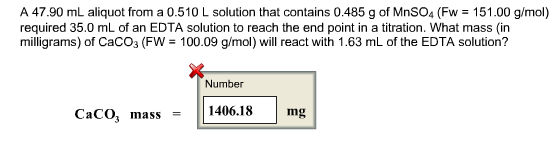explanation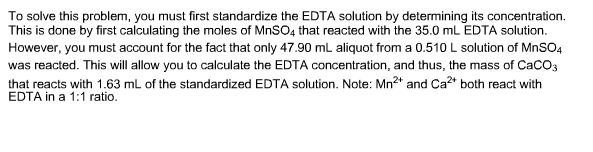A 47.90 mL aliquot from a 0.510 L solution that contains 0.485 g of MnSO4 (Fw 151.00 g/mol) required 35.0 mL of an EDTA solution to reach the end point in a titration. What mass (in milligrams) of CaCO3 (FW 100.09 g/mol) will react with 1.63 m of the EDTA solution? Number Caco, mass 1406.18 mg

#### Homework Answers

Answer #1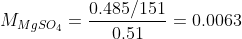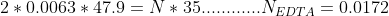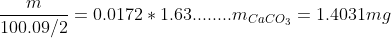Know the answer?
Your Answer:

#### Post as a guest

Your Name:

What's your source?

#### Earn Coin

Coins can be redeemed for fabulous gifts.

Not the answer you're looking for? Ask your own homework help question. Our experts will answer your question WITHIN MINUTES for Free.
Similar Homework Help Questions
• ### A 47.30 mL aliquot from a 0.485 L solution that contains 0.465 g of MnSO4 (MW=151.00...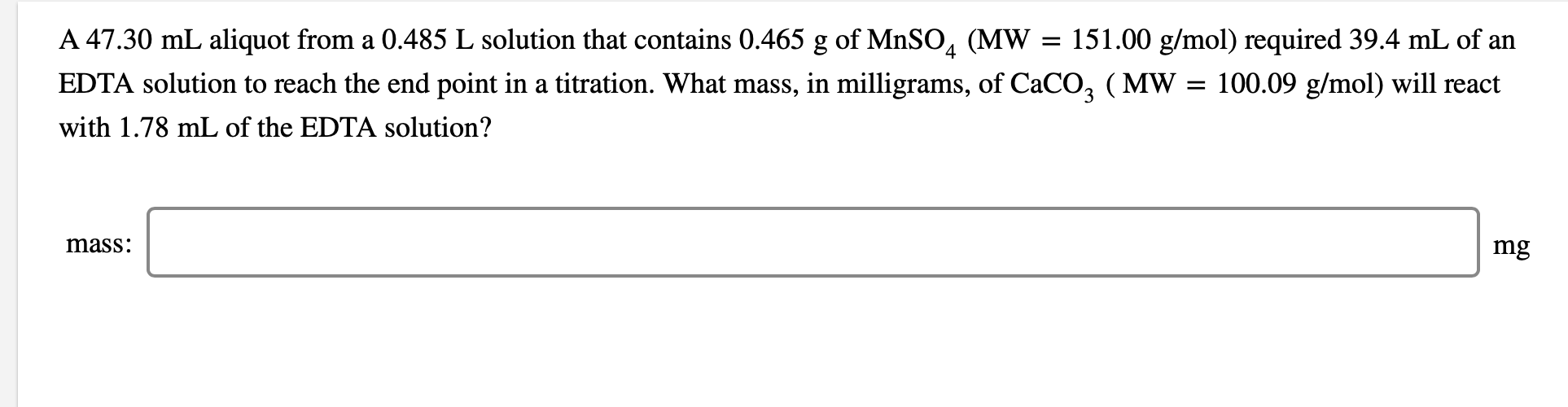A 47.30 mL aliquot from a 0.485 L solution that contains 0.465 g of MnSO4 (MW=151.00 g/mol) required 39.4 mL of an EDTA solution to reach the end point in a titration. What mass, in milligrams, of CaCO3 ( MW=100.09 g/mol) will react with 1.78 mL of the EDTA solution? A 47.30 mL aliquot from a 0.485 L solution that contains 0.465 g of MnSO4 (MW = 151.00 g/mol) required 39.4 mL of an EDTA solution to reach the end...

• ### A 50.00 mL aliquot from a 0.490 L solution that contains 0.470 g of MnSO4 (...

A 50.00 mL aliquot from a 0.490 L solution that contains 0.470 g of MnSO4 ( MW=151.00 g/mol) required 38.0 mL of an EDTA solution to reach the end point in a titration. What mass, in milligrams, of CaCO3 ( MW=100.09 g/mol) will react with 1.00 mL of the EDTA solution? mass: mg

• ### A 48.80 mL aliquot from a 0.505 L solution that contains 0.525 g of MnSOg (MW...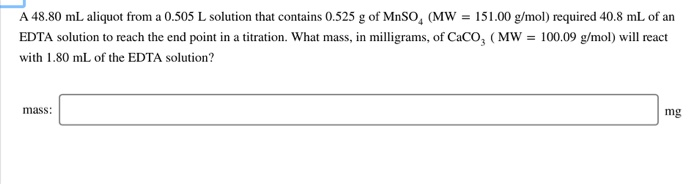A 48.80 mL aliquot from a 0.505 L solution that contains 0.525 g of MnSOg (MW EDTA solution to reach the end point in a titration. What mass, in milligrams, of CaCo3 (MW 100.09 g/mol) will react 151.00 g/mol) required 40.8 mL of an with 1.80 mL of the EDTA solution? mass mg

• ### A 48.30 mL aliquot from a 0.460 L solution that contains 0.375 g of MnSO, (MW = 151.00 g/mol) required 39.9 mL of an ED...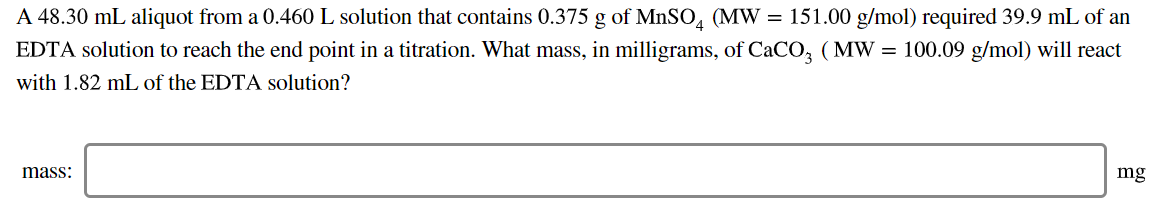A 48.30 mL aliquot from a 0.460 L solution that contains 0.375 g of MnSO, (MW = 151.00 g/mol) required 39.9 mL of an EDTA solution to reach the end point in a titration. What mass, in milligrams, of CaCO, (MW = 100.09 g/mol) will react with 1.82 mL of the EDTA solution? mass: mg

• ### Please help! Will rate. Cd" forms two complexes with acetate, with the given overall formation constants....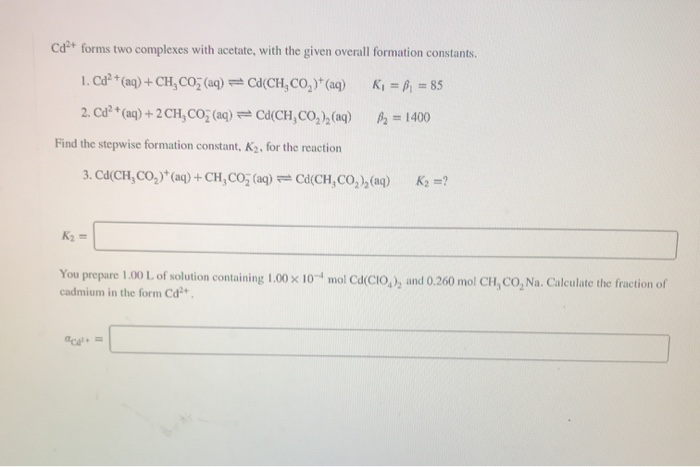Please help! Will rate. Cd" forms two complexes with acetate, with the given overall formation constants. 1. Cd2+ (aq) + CH, COŽ (aq) =C(CH.CO2)+(aq) 2. Cd²+ (aq) + 2CH,CO2 (aq) = CaCH,CO2),(aq) R = B = 85 B2 = 1400 Find the stepwise formation constant, K2, for the reaction 3. Cd(CH, CO)* (aq) +CHCO, (aq) = Ca(CH, CO ) (aq) Kg =? You prepare 1.00L of solution containing 1.00 x 10-mol Ca(CIO), and 0.260 mol CH, CO, Na. Calculate the...

• ### Name Section Experiment 28 Advance Study Assignment: Determination of the Hardness of Water 1. A 0.3946...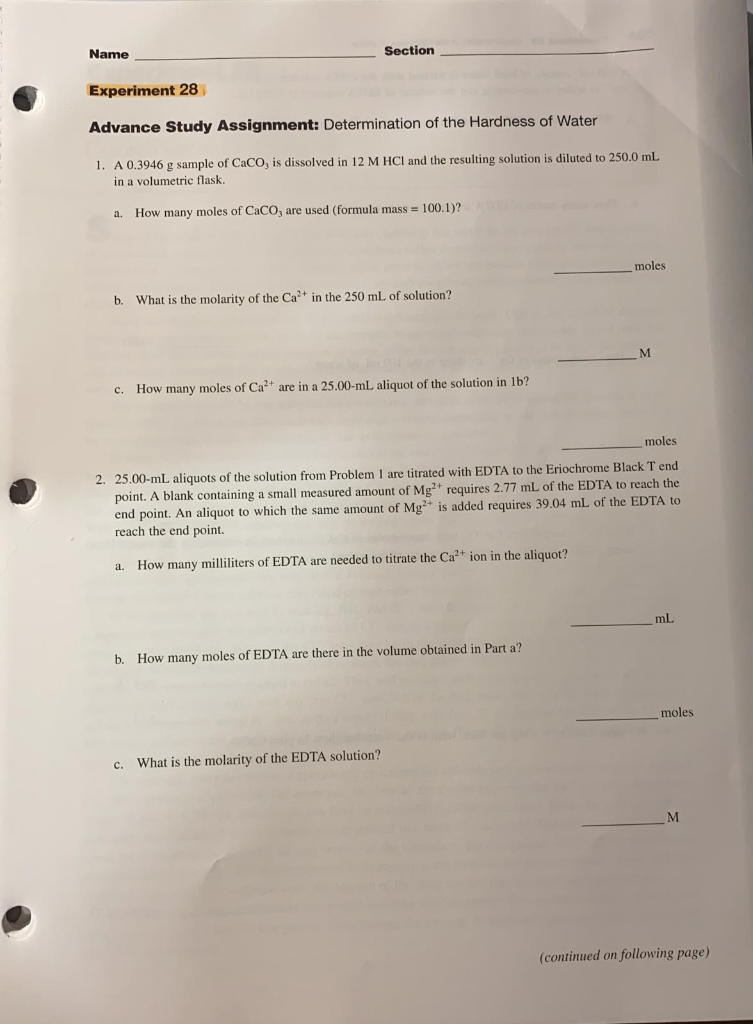Name Section Experiment 28 Advance Study Assignment: Determination of the Hardness of Water 1. A 0.3946 g sample of Caco, is dissolved in 12 M HCl and the resulting solution is diluted to 250.0 mL in a volumetric flask. a. How many moles of Caco, are used (formula mass = 100.1)? moles b. What is the molarity of the Ca2+ in the 250 mL of solution? c. How many moles of Caare in a 25.00-mL aliquot of the solution in...

• ### A solution contains 1.577 mg of CoSO4 (155.0 g/mol) per milliliter. Calculate a. the volume of...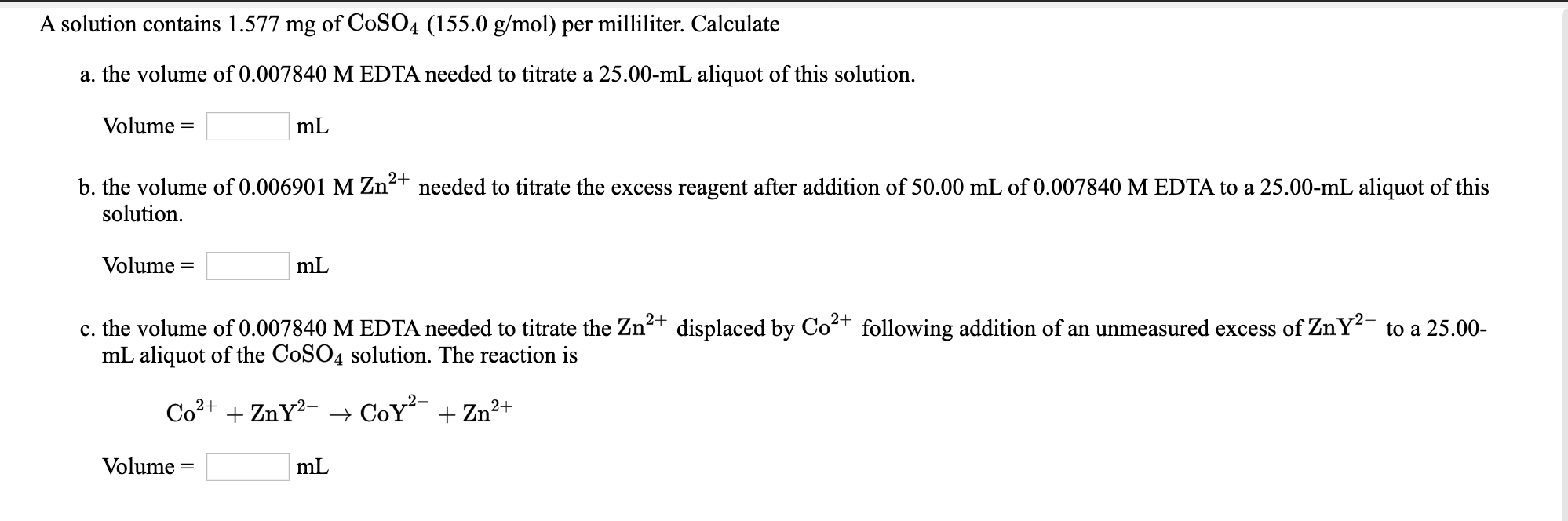A solution contains 1.577 mg of CoSO4 (155.0 g/mol) per milliliter. Calculate a. the volume of 0.007840 M EDTA needed to titrate a 25.00-mL aliquot of this solution. Volume = mL b. the volume of 0.006901 M Zn2+ needed to titrate the excess reagent after addition of 50.00 mL of 0.007840 M EDTA to a 25.00-mL aliquot of this solution. Volume = mL c. the volume of 0.007840 M EDTA needed to titrate the Zn2+ displaced by Co2+ following addition...

• ### Co. A 50.0 mL aliquot of water (assume contains only Ca?") required 4.08 ml. of o.0100...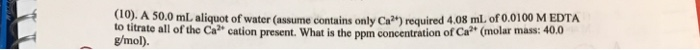Co. A 50.0 mL aliquot of water (assume contains only Ca?") required 4.08 ml. of o.0100 M EDTA to titrate all of the Cr" cation present what is the ppm concentration of Ca2+ (molar mass: 400 g/mol).

• ### . A 10.00-ml aliquot of vinegar requires 16.95 mL of the 0.4874 M standa point of...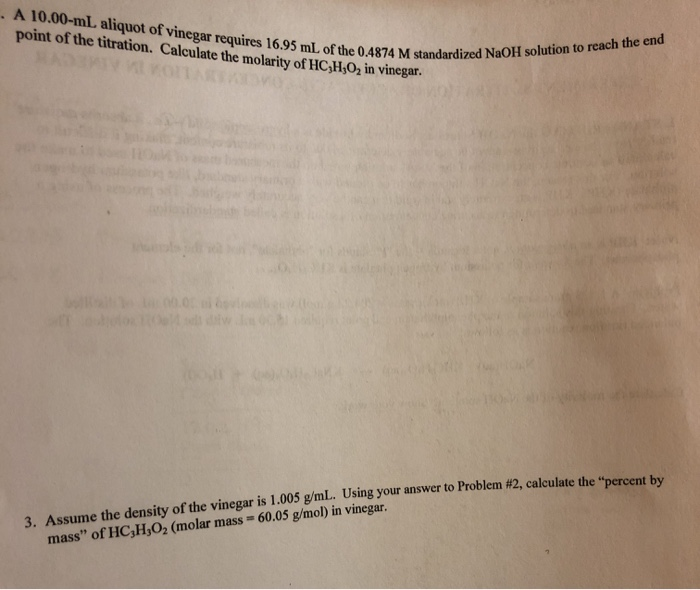. A 10.00-ml aliquot of vinegar requires 16.95 mL of the 0.4874 M standa point of the titration. Calculate the molarity of HC,H,O, in vinegar. 4 M standardized NaOH solution to reach the end w swer to Problem #2, calculate the "percent by 3. Assume the density of the vinegar is 1.005 g/mL. Using your answer to Problem #2, calculate th mass" of HC,H,O2 (molar mass = 60.05 g/mol) in vinegar.

• ### A student needs to prepare 100.0 mL of vitamin C solution according to the directions in...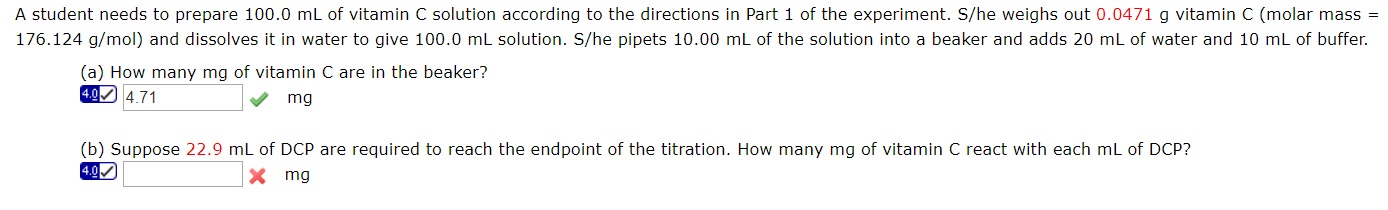A student needs to prepare 100.0 mL of vitamin C solution according to the directions in Part 1 of the experiment. S/he weighs out 0.0471 g vitamin C (molar mass = 176.124 g/mol) and dissolves it in water to give 100.0 ml solution. S/he pipets 10.00 mL of the solution into a beaker and adds 20 mL of water and 10 mL of buffer. (a) How many mg of vitamin C are in the beaker? 4.9 4.71 mg (b) Suppose...

Free Homework App

Scan Your Homework
to Get Instant Free Answers
Need Online Homework Help?

Get Answers For Free
Most questions answered within 3 hours.